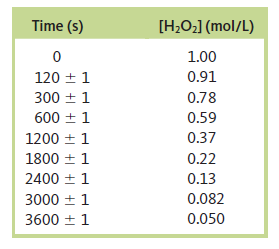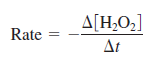# Problem: A possible mechanism for the decomposition of hydrogen peroxide isH2O2 → 2OHH2O2 + OH → H2O + HO2HO2 + OH → H2O + O2Using your results from Exercise 37, specify which step is the rate-determining step. What is the overall balanced equation for the reaction?---------------------Exercise 37. The decomposition of hydrogen peroxide was studied, and the following data were obtained at a particular temperature:Assuming thatdetermine the rate law, the integrated rate law, and the value of the rate constant. Calculate [H2O2] at 4000. s after the start of the reaction.

###### FREE Expert Solution

For this problem, we are being asked to write the overall balanced equation for the reaction

H2O→ 2OH
H2O2 + OH → H2O + HO2
HO2 + OH → H2O + O2

In a given reaction mechanism, overall reaction can be determined by adding the given reactions:

79% (189 ratings)###### Problem Details

A possible mechanism for the decomposition of hydrogen peroxide is

H2O→ 2OH
H2O2 + OH → H2O + HO2
HO2 + OH → H2O + O2

Using your results from Exercise 37, specify which step is the rate-determining step. What is the overall balanced equation for the reaction?

---------------------

Exercise 37. The decomposition of hydrogen peroxide was studied, and the following data were obtained at a particular temperature:Assuming thatdetermine the rate law, the integrated rate law, and the value of the rate constant. Calculate [H2O2] at 4000. s after the start of the reaction.

Frequently Asked Questions

What scientific concept do you need to know in order to solve this problem?

Our tutors have indicated that to solve this problem you will need to apply the Reaction Mechanism concept. If you need more Reaction Mechanism practice, you can also practice Reaction Mechanism practice problems.

What professor is this problem relevant for?

Based on our data, we think this problem is relevant for Professor Kobko-Litskevitch's class at HUNTER.

What textbook is this problem found in?

Our data indicates that this problem or a close variation was asked in Chemistry: An Atoms First Approach - Zumdahl Atoms 1st 2nd Edition. You can also practice Chemistry: An Atoms First Approach - Zumdahl Atoms 1st 2nd Edition practice problems.